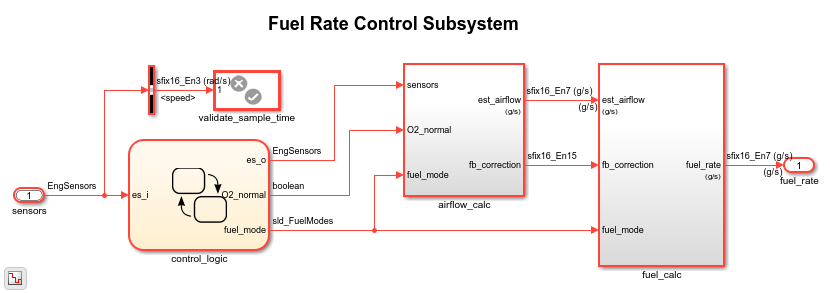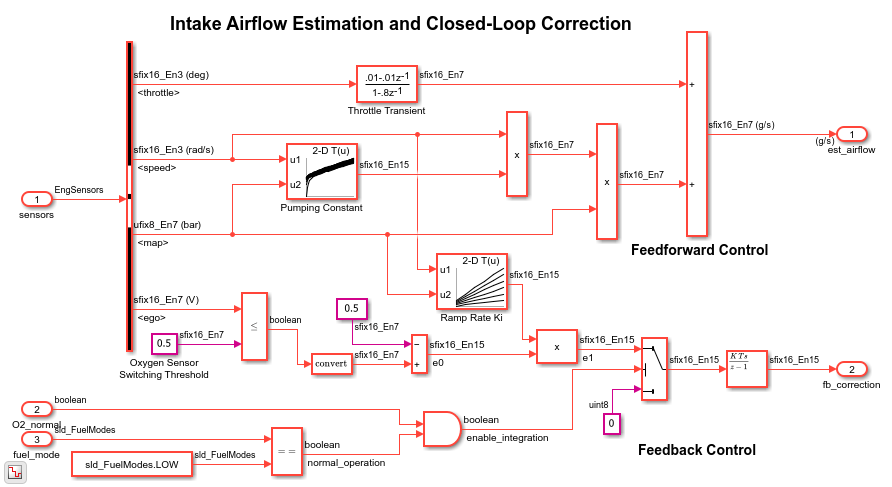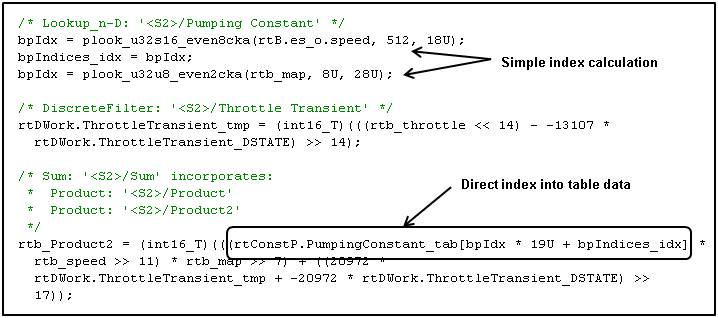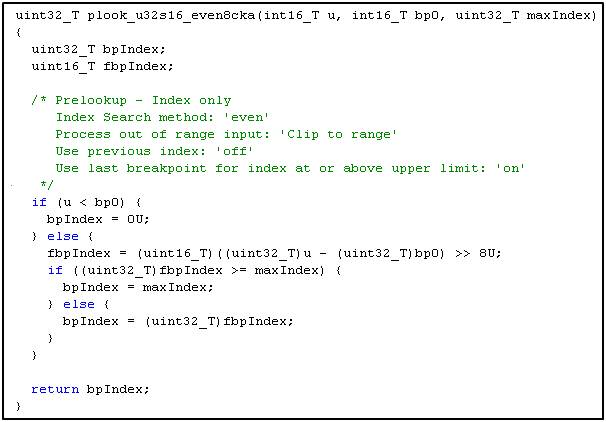# Air-Fuel Ratio Control System with Fixed-Point Data

This example shows how to generate and optimize the code for a fixed-point air-fuel ratio control system designed with Simulink® and Stateflow®. For a detailed explanation of the model, see:

The example uses the Embedded Coder® system target file (`ert.tlc`).

### Relevant Portions of the Model

Figures 1-4 show relevant portions of the `sldemo_fuelsys` model, which is a closed-loop system containing a plant subsystem and a controller subsystem. The plant allows engineers to validate the controller through simulation early in the design cycle. In this example, generate code for the relevant controller subsystem, `fuel_rate_control`. Figure 1 shows the top-level simulation model.

```% Open |sldemo_fuelsys|, set the parameters and update the model diagram % to view the signal data types. close_system('sldemo_fuelsys',0) load_system('sldemo_fuelsys'); rtwconfiguredemo('sldemo_fuelsys','ERT','fixed'); sldemo_fuelsys_data('sldemo_fuelsys','switch_data_type','fixed'); sldemo_fuelsys_data('sldemo_fuelsys','set_info_text','rtwdemo_fuelsys_fxp_script'); sldemo_fuelsys_data('sldemo_fuelsys','top_level_logging','on'); set_param('sldemo_fuelsys','ShowPortDataTypes','on'); set_param('sldemo_fuelsys','SampleTimeColors','on'); set_param('sldemo_fuelsys','Dirty','off'); sldemo_fuelsys([],[],[],'compile'); sldemo_fuelsys([],[],[],'term'); ```Figure 1: Top-level model of the plant and controller

The air-fuel ratio control system is composed of Simulink® and Stateflow® blocks. It is the portion of the model for which to generate code.

```open_system('sldemo_fuelsys/fuel_rate_control'); ```Figure 2: The air-fuel ratio controller subsystem

The intake airflow estimation and closed loop correction system contains two lookup tables, Pumping Constant and Ramp Rate Ki.

```open_system('sldemo_fuelsys/fuel_rate_control/airflow_calc'); ```Figure 3: The airflow_calc subsystem

The control logic is a Stateflow® chart that specifies the different modes of operation.

```open_system('sldemo_fuelsys/fuel_rate_control/control_logic'); ```Figure 4: Fuel ratio controller logic

Remove the window clutter.

```close_system('sldemo_fuelsys/fuel_rate_control/airflow_calc'); close_system('sldemo_fuelsys/fuel_rate_control/fuel_calc'); close_system('sldemo_fuelsys/fuel_rate_control/control_logic'); hDemo.rt=sfroot;hDemo.m=hDemo.rt.find('-isa','Simulink.BlockDiagram'); hDemo.c=hDemo.m.find('-isa','Stateflow.Chart','-and','Name','control_logic'); hDemo.c.visible=false; close_system('sldemo_fuelsys/fuel_rate_control'); ```

Build the air-fuel ratio control system only. Once the code generation process is complete, an HTML report detailing the generated code is displayed. The main body of the code is located in `fuel_rate_control.c`.

```slbuild('sldemo_fuelsys/fuel_rate_control'); ```
```### Starting build procedure for: fuel_rate_control ### Successful completion of build procedure for: fuel_rate_control Build Summary Top model targets built: Model Action Rebuild Reason ================================================================================================== fuel_rate_control Code generated and compiled Code generation information file does not exist. 1 of 1 models built (0 models already up to date) Build duration: 0h 0m 25.349s ```

Figure 5 shows snippets of the generated code for the lookup table Pumping Constant.

To see the code for Pumping Constant, right-click the block and select C/C++ Code > Navigate To C/C++ Code.

```rtwtrace('sldemo_fuelsys/fuel_rate_control/airflow_calc/Pumping Constant'); ```

The code for the pumping constant contains two breakpoint searches and a 2D interpolation. The `SpeedVect` breakpoint is unevenly spaced, and while the `PressVect` breakpoint is evenly spaced, neither have power of two spacing. The current spacing leads to extra code (ROM), including a division, and requires all breakpoints to be in memory (RAM).Figure 5: Generated code for Pumping Constant lookup (contains unevenly spaced breakpoints)

### Optimize Code with Evenly Spaced Power of Two Breakpoints

You can optimize the generated code performance by using evenly spaced power of two breakpoints. In this example, remap the lookup table data in the air-fuel ratio control system based on the existing measured data.

When you loaded the model, the model `PostLoadFcn` created the lookup table data in the model workspace. Retrieve the original table data via `sldemo_fuelsys_data`, modify it for evenly spaced power of two, and reassign it in the model workspace.

```td = sldemo_fuelsys_data('sldemo_fuelsys', 'get_table_data'); ```

Compute new table data for evenly spaced power of two breakpoints.

```ntd.SpeedVect = 64 : 2^5 : 640; % 32 rad/sec ntd.PressVect = 2*2^-5 : 2^-5 : 1-(2*2^-5); % 0.03 bar ntd.ThrotVect = 0:2^1:88; % 2 deg ntd.RampRateKiX = 128:2^7:640; % 128 rad/sec ntd.RampRateKiY = 0:2^-2:1; % 0.25 bar ```

Remap table data.

```ntd.PumpCon = interp2(td.PressVect,td.SpeedVect,td.PumpCon, ntd.PressVect',ntd.SpeedVect); ntd.PressEst = interp2(td.ThrotVect,td.SpeedVect,td.PressEst,ntd.ThrotVect',ntd.SpeedVect); ntd.SpeedEst = interp2(td.PressVect,td.ThrotVect,td.SpeedEst,ntd.PressVect',ntd.ThrotVect); ntd.ThrotEst = interp2(td.PressVect,td.SpeedVect,td.ThrotEst,ntd.PressVect',ntd.SpeedVect); ```

Recompute Ramp Rate table data.

```ntd.RampRateKiZ = (1:length(ntd.RampRateKiX))' * (1:length(ntd.RampRateKiY)) * td.Ki; ```

### Pumping Constant Power Of Two Spacing

```figure('Tag','CloseMe'); mesh(td.PressVect,td.SpeedVect,td.PumpCon), hold on mesh(ntd.PressVect,ntd.SpeedVect,ntd.PumpCon) xlabel('PressVect'), ylabel('SpeedVect'), zlabel('PumpCon') title(sprintf('Pumping Constant\nOriginal Spacing (%dx%d) vs. Power of Two Spacing (%dx%d)',... size(td.PumpCon,1),size(td.PumpCon,2),size(ntd.PumpCon,1),size(ntd.PumpCon,2))); ```### Pressure Estimate Power Of Two Spacing

```clf mesh(td.ThrotVect,td.SpeedVect,td.PressEst), hold on mesh(ntd.ThrotVect,ntd.SpeedVect,ntd.PressEst) xlabel('ThrotVect'), ylabel('SpeedVect'), zlabel('PressEst') title(sprintf('Pressure Estimate\nOriginal Spacing (%dx%d) vs. Power of Two Spacing (%dx%d)',... size(td.PressEst,1),size(td.PressEst,2),size(ntd.PressEst,1),size(ntd.PressEst,2))); ```### Speed Estimate Power Of Two Spacing

```clf mesh(td.PressVect,td.ThrotVect,td.SpeedEst), hold on, mesh(ntd.PressVect,ntd.ThrotVect,ntd.SpeedEst) xlabel('PressVect'), ylabel('ThrotVect'), zlabel('SpeedEst') title(sprintf('Speed Estimate\nOriginal Spacing (%dx%d) vs. Power of Two Spacing (%dx%d)',... size(td.SpeedEst,1),size(td.SpeedEst,2),size(ntd.SpeedEst,1),size(ntd.SpeedEst,2))); ```### Throttle Estimate Power Of Two Spacing

```clf mesh(td.PressVect,td.SpeedVect,td.ThrotEst), hold on mesh(ntd.PressVect,ntd.SpeedVect,ntd.ThrotEst) xlabel('PressVect'), ylabel('SpeedVect'), zlabel('ThrotEst') title(sprintf('Throttle Estimate\nOriginal Spacing (%dx%d) vs. Power of Two Spacing (%dx%d)',... size(td.ThrotEst,1),size(td.ThrotEst,2),size(ntd.ThrotEst,1),size(ntd.ThrotEst,2))); ```### Ramp Rate Ki Power Of Two Spacing

```clf mesh(td.RampRateKiX,td.RampRateKiY,td.RampRateKiZ'), hold on mesh(ntd.RampRateKiX,ntd.RampRateKiY,ntd.RampRateKiZ'), hidden off xlabel('RampRateKiX'), ylabel('RampRateKiY'), zlabel('RampRateKiZ') title(sprintf('Ramp Rate Ki\nOriginal Spacing (%dx%d) vs. Power of Two Spacing (%dx%d)',... size(td.RampRateKiZ,1),size(td.RampRateKiZ,2),size(ntd.RampRateKiZ,1),size(ntd.RampRateKiZ,2))); ```The default configuration causes the model to log simulation data for the top-level signals. These simulation results are stored in the workspace variable `sldemo_fuelsys_output`. Before updating the model workspace with the new data, save the result of the simulation in `hDemo.orig_data` for later comparison with the evenly spaced power of two table simulation.

```set_param('sldemo_fuelsys','StopTime','8') sim('sldemo_fuelsys') hDemo.orig_data = sldemo_fuelsys_output; ```

Reassign the new table data in the model workspace.

```hDemo.hWS = get_param('sldemo_fuelsys', 'ModelWorkspace'); hDemo.hWS.assignin('PressEst', ntd.PressEst); hDemo.hWS.assignin('PressVect', ntd.PressVect); hDemo.hWS.assignin('PumpCon', ntd.PumpCon); hDemo.hWS.assignin('SpeedEst', ntd.SpeedEst); hDemo.hWS.assignin('SpeedVect', ntd.SpeedVect); hDemo.hWS.assignin('ThrotEst', ntd.ThrotEst); hDemo.hWS.assignin('ThrotVect', ntd.ThrotVect); hDemo.hWS.assignin('RampRateKiX',ntd.RampRateKiX); hDemo.hWS.assignin('RampRateKiY',ntd.RampRateKiY); hDemo.hWS.assignin('RampRateKiZ',ntd.RampRateKiZ); ```

Reconfigure lookup tables for evenly spaced data.

```hDemo.lookupTables = find_system(get_param('sldemo_fuelsys','Handle'),... 'BlockType','Lookup_n-D'); for hDemo_blkIdx = 1 : length(hDemo.lookupTables) hDemo.blkH = hDemo.lookupTables(hDemo_blkIdx); set_param(hDemo.blkH,'IndexSearchMethod','Evenly spaced points') set_param(hDemo.blkH,'InterpMethod','None - Flat') set_param(hDemo.blkH,'ProcessOutOfRangeInput','None') end ```

Rerun the simulation for the evenly spaced power of two implementation, and store the result of the simulation in `hDemo.pow2_data`.

```sim('sldemo_fuelsys') hDemo.pow2_data = sldemo_fuelsys_output; ```

Compare the result of the simulation for the fuel flow rate and the air fuel ratio. The simulation exercised the Pumping Constant and Ramp Rate Ki lookup tables, and shows an excellent match for the evenly spaced power of two breakpoints relative to the original table data.

```figure('Tag','CloseMe'); subplot(2,1,1); plot(hDemo.orig_data.get('fuel').Values.Time, ... hDemo.orig_data.get('fuel').Values.Data,'r-'); hold plot(hDemo.pow2_data.get('fuel').Values.Time, ... hDemo.pow2_data.get('fuel').Values.Data,'b-'); ylabel('FuelFlowRate (g/sec)'); title('Fuel Control System: Table Data Comparison'); legend('original','even power of two'); axis([0 8 .75 2.25]); subplot(2,1,2); plot(hDemo.orig_data.get('air_fuel_ratio').Values.Time, ... hDemo.orig_data.get('air_fuel_ratio').Values.Data,'r-'); hold plot(hDemo.pow2_data.get('air_fuel_ratio').Values.Time, ... hDemo.pow2_data.get('air_fuel_ratio').Values.Data,'b-'); ylabel('Air/Fuel Ratio'); xlabel('Time (sec)'); legend('original','even power of 2','Location','SouthEast'); axis([0 8 11 16]); ```
```Current plot held Current plot held ```Rebuild the air-fuel ratio control system and compare the difference in the generated lookup table code.

```slbuild('sldemo_fuelsys/fuel_rate_control'); ```
```### Starting build procedure for: fuel_rate_control ### Successful completion of build procedure for: fuel_rate_control Build Summary Top model targets built: Model Action Rebuild Reason ================================================================================= fuel_rate_control Code generated and compiled Generated code was out of date. 1 of 1 models built (0 models already up to date) Build duration: 0h 0m 21.114s ```

Figure 6 shows the same snippets of the generated code for the 'Pumping Constant' lookup table. The generated code for evenly spaced power of two breakpoints is significantly more efficient than the unevenly spaced breakpoint case. The code consists of two simple breakpoint calculations and a direct index into the 2D lookup table data. The expensive division is avoided and the breakpoint data is not required in memory.

```rtwtrace('sldemo_fuelsys/fuel_rate_control/airflow_calc/Pumping Constant'); ```Figure 6: Generated code for Pumping Constant lookup (evenly spaced power of two breakpoints)

Close the example.

```close(findobj(0,'Tag','CloseMe')); clear hDemo* td ntd close_system('sldemo_fuelsys',0); rtwdemoclean; ```

### Model Advisor for Code Efficiency

Improving code efficiency by using evenly spaced power of two breakpoints is one of several important optimizations for fixed-point code generation. The Simulink® Model Advisor is a great tool for identifying other methods of improving code efficiency for a Simulink® and Stateflow® model. Make sure to run the checks under the Embedded Coder® folder or Simulink Coder®.